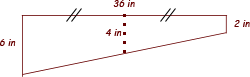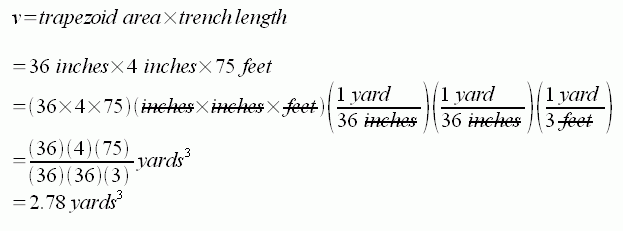SEARCH HOMEMath Central Quandaries & QueriesQuestion from mark, a parent: We need to fill in dirt along a 75 foot long fence, 3 feet out , statring at 6 inches agaisnt the fence, sloping to 2 inches at the grass. I am tryign to calculate the volume to order enough cubic yards of dirt to fill this. Please helpHi Mark.

If I understand correctly, you are looking for the wedge-shaped volume in this diagram:Where it is actually 75 ft long, 3 feet horizontally and between 6 and 2 inches deep. Thus, the cross section looks like this:This shape is a trapezoid (in the UK it is called a trapezium). The area of this trapezoid times the length of the trench will be the volume of the fill you need.

The area of a trapezoid is simply the distance between the parallel sides times the midpoint distance. Thus, in your case, it is 36 inches times 4 inches.

So the volume of your wedge is this:So that's how much dirt you'll need to fill the trench.

Stephen La Rocque. >Math Central is supported by the University of Regina and The Pacific Institute for the Mathematical Sciences.